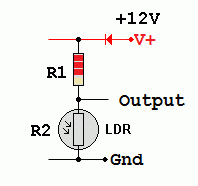RANDOM PAGE

SITE SEARCH

LOG
IN

HELP

# Light Dependent Resistor

This is the AQA version closing after June 2019. Visit the the version for Eduqas instead.

## LDR Properties

The LDR Resistance decreases with increasing light intensity.• LDRs are used to measure the light level.
• They are slow to react so they are not used with fibre-optic or other optical communications systems.
• They are used in light meters for photography and for systems that turn on lights when it gets dark.
• The LDR is normally used with a fixed resistor. This prevents too much current flowing when the light is very bright.
• The LDR and fixed resistor convert the varying light level into a varying voltage.

## LDR Protection

The LDR is wired up with a resistor in series.
This makes a voltage divider circuit.
The series resistor is chosen to satisfy two
design constraints.

• When the light is bright and the LDR
resistance drops close to zero, the other
resistor MUST ensure that the LDR
current remains below a safe limit.
• It is also desirable if the LDR
resistance and the series resistance
are approximately equal at the light
level you are trying to detect.

### Calculate the Safety Resistor

Assume the LDR resistance is small in very
bright light. In a 12 Volt circuit, if the maximum
safe current is 10 mA ...

R = V / I      R = 12 / 0.01      R = 1.2KΩ

## Voltage DividerVout = 12 x R2 / ( R1 + R2 )

As the light gets brighter, R2
decreases and the output
voltage drops.

## Voltage DividerVout = 12 x R2 / ( R1 + R2 )

As the light gets brighter, R1
decreases and the output
voltage rises.

## How the LDR Works

Light Dependent Resistors (LDR) are made from a semiconductor. In the dark, this semiconductor has very few free carriers so the LDR has a high resistance. When photons of light hit the semiconductor, they add enough energy to dislodge carriers which can then flow. This reduces the resistance. The carriers are electrons.

## Logarithmic Graphs

As the light level changes, the resistance of the LDR changes over a huge range. This is difficult to plot onto normal graph paper so logarithmic graphs are used.• If the resistance is 20kΩ, the light level is about 0.42 Lux.
• If the resistance is 3kΩ, the light level is 20 Lux.
• If the light level is 3 Lux, the resistance is 2.7kΩ.
• If the light level is 600 Lux, the resistance is about 10Ω which is close to zero.

## Calculating the Safety Resistor

• For this LDR, the absolute maximum current is 50mA.
• In bright light, assume the LDR resistance drops to zero.

If the LDR is in a 12 volt circuit, the series resistor must be at least ...

R = V / I

R = 12 / 50x10-3

R = 240Ω

240Ω is the minimum safe resistance.

If the goal is to detect a light level less than 10 Lux, a more suitable resistor would be about 800Ω.

This resistor satisfies both the design requirements mentioned at the top of this page.